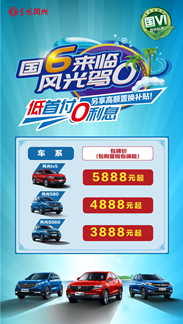## 国六来临 东风风光至高优惠14000元

2019年07月09日 18:18 来源：车行天下 超过：14701次关注

7月1日起，机动车国六标准开始实施，中国汽车市场正式跨入“国六”时代。凭借雄厚的技术实力，东风风光率先完成国六更新，现东风风光全系国六车型已经上市。更让人心动的是，为了让消费者尽早体验高品质国六车型，东风风光特推出“低首付0息购”大型盛夏购车钜惠行动，全系金融补贴至高可达8000元，置换补贴至高可达6000元，两者可叠加享受至高14000元，还有终身免费流量和超级质保大礼包等优惠豪礼，同时用户只需支付888元起，将免受上牌等繁琐手续将新车带牌开回家。低首付，圆你购车梦

作为自主品牌，东风风光在产品上紧紧把握行业发展趋势和消费潮流，持续打造颇具竞争力的产品。此次“国六”政策发布，东风风光更是走在一众车企的前面，将东风风光全系车型进行了全方位升级，为用户带来全新的优质产品体验，并送上诚意满满的购车钜惠。

活动期间，东风风光明星产品全线出击，钜惠空前，全系享受低首付0利息金融服务，让广大用户们提前开上“国六”新车。其中，风光ix5，金融补贴至高可达8000元，最低首付仅需5888元;风光580，金融补贴至高可达4000元，最低首付只要4888元;风光S560，金融补贴至高可达2500元，最低首付低至3888元。特别礼遇，钜惠送豪礼

不仅如此，为了回馈老车主们一直以来对东风风光的支持与信赖，活动期间，凡是东风风光车主以及直系亲属，如父母、子女、配偶，换购或增购风光品牌新车，东风风光将特别送出价值最高6000元的现金和保养双重大礼。

同时，东风风光老车主介绍新客户成功购车，还可享受感恩三重礼：到店礼、保养礼和邂逅礼。其中到店礼为风迷中的硬通货“风迷币”，保养礼为含四十项爱车检查、小保养，工时费免费送，推荐越多次数越多。还有独特的邂逅礼，车主与推荐朋友每人可获得一张价值1880元的“东风风光畅游七彩云南礼券”，四天三夜免费住宿以及客房礼让您宾至如归，专车接送机服务，伴手礼及景区优惠券给您贵宾级的礼遇，让车友与朋友一路嗨玩更带感。

此次活动促销力度之大，优惠范围之广可谓史无前例。而在产品服务上，东风风光更有汽车行业中具有竞争优势的“7年/15万公里超级质保”等诚意满满的质保服务，用户可享受至高价值6000元的质保大礼包，还有价值3000元的终身免费流量福利，不仅让用户轻松购车，更让用户用车无忧。

家不需要大，温馨最好，车不需豪华，装下生活的梦想就成。面对如此高性价比的选择，你是不是很想入手呢，快快响应钜惠活动的“呼唤”，将东风风光“国六”新车开回家吧。

#### 相关文章

0-500 字已有评论 0条 查看评论>>

### 热门标签

﻿
• 快速找车
• 选择品牌
• 选择品牌
• A  奥迪
• A  阿斯顿·马丁
• A  阿尔法·罗密欧
• B  宝沃
• B  布加迪
• B  巴博斯
• B  保时捷
• B  宾利
• B  奔驰
• B  宝马
• B  本田
• B  别克
• B  标致
• B  比亚迪
• B  宝骏
• B  北汽制造
• B  北汽新能源
• B  北汽幻速
• B  北汽威旺
• B  北京汽车
• B  奔腾
• B  北汽绅宝
• C  长安
• C  长安商用
• C  长城
• C  昌河
• D  大众
• D  道奇
• D  DS
• D  东南
• D  东风风神
• D  东风风行
• D  东风小康
• D  东风风度
• D  东风
• F  福特
• F  丰田
• F  菲亚特
• F  法拉利
• F  福田
• F  福迪
• F  福汽启腾
• G  观致
• G  广汽传祺
• G  广汽吉奥
• G  GMC
• H  红旗
• H  汉腾汽车
• H  哈弗
• H  哈飞
• H  海格
• H  海马
• H  华颂
• H  黄海
• H  华泰
• H  恒天
• J  吉利汽车
• J  捷豹
• J  Jeep
• J  江淮
• J  江铃
• J  金杯
• J  九龙
• J  金旅
• K  凯翼
• K  凯迪拉克
• K  克莱斯勒
• K  科尼塞克
• K  卡威
• K  开瑞
• L  路虎
• L  林肯
• L  劳斯莱斯
• L  兰博基尼
• L  雷克萨斯
• L  铃木
• L  雷诺
• L  理念
• L  力帆
• L  莲花汽车
• L  猎豹
• L  路特斯
• L  陆风
• M  马自达
• M  MG
• M  MINI
• M  玛莎拉蒂
• M  摩根
• M  迈凯轮
• N  纳智捷
• O  欧宝
• O  讴歌
• O  欧朗
• Q  奇瑞
• Q  起亚
• Q  启辰
• R  日产
• R  荣威
• R  瑞麒
• S  三菱
• S  斯威汽车
• S  萨博
• S  smart
• S  斯柯达
• S  斯巴鲁
• S  思铭
• S  双龙
• S  上汽大通
• S  双环
• T  特斯拉
• T  腾势
• W  沃尔沃
• W  五菱汽车
• W  五十铃
• W  威兹曼
• W  威麟
• X  现代
• X  雪佛兰
• X  雪铁龙
• X  西雅特
• Y  一汽
• Y  英菲尼迪
• Y  英致
• Y  依维柯
• Y  野马汽车
• Y  永源
• Z  众泰
• Z  中华
• Z  中兴
• Z  知豆
• 选择车系
• 选择车系
• 车型对比
• 选择品牌
• 选择品牌
• A  奥迪
• A  阿斯顿·马丁
• A  阿尔法·罗密欧
• B  宝沃
• B  布加迪
• B  巴博斯
• B  保时捷
• B  宾利
• B  奔驰
• B  宝马
• B  本田
• B  别克
• B  标致
• B  比亚迪
• B  宝骏
• B  北汽制造
• B  北汽新能源
• B  北汽幻速
• B  北汽威旺
• B  北京汽车
• B  奔腾
• B  北汽绅宝
• C  长安
• C  长安商用
• C  长城
• C  昌河
• D  大众
• D  道奇
• D  DS
• D  东南
• D  东风风神
• D  东风风行
• D  东风小康
• D  东风风度
• D  东风
• F  福特
• F  丰田
• F  菲亚特
• F  法拉利
• F  福田
• F  福迪
• F  福汽启腾
• G  观致
• G  广汽传祺
• G  广汽吉奥
• G  GMC
• H  红旗
• H  汉腾汽车
• H  哈弗
• H  哈飞
• H  海格
• H  海马
• H  华颂
• H  黄海
• H  华泰
• H  恒天
• J  吉利汽车
• J  捷豹
• J  Jeep
• J  江淮
• J  江铃
• J  金杯
• J  九龙
• J  金旅
• K  凯翼
• K  凯迪拉克
• K  克莱斯勒
• K  科尼塞克
• K  卡威
• K  开瑞
• L  路虎
• L  林肯
• L  劳斯莱斯
• L  兰博基尼
• L  雷克萨斯
• L  铃木
• L  雷诺
• L  理念
• L  力帆
• L  莲花汽车
• L  猎豹
• L  路特斯
• L  陆风
• M  马自达
• M  MG
• M  MINI
• M  玛莎拉蒂
• M  摩根
• M  迈凯轮
• N  纳智捷
• O  欧宝
• O  讴歌
• O  欧朗
• Q  奇瑞
• Q  起亚
• Q  启辰
• R  日产
• R  荣威
• R  瑞麒
• S  三菱
• S  斯威汽车
• S  萨博
• S  smart
• S  斯柯达
• S  斯巴鲁
• S  思铭
• S  双龙
• S  上汽大通
• S  双环
• T  特斯拉
• T  腾势
• W  沃尔沃
• W  五菱汽车
• W  五十铃
• W  威兹曼
• W  威麟
• X  现代
• X  雪佛兰
• X  雪铁龙
• X  西雅特
• Y  一汽
• Y  英菲尼迪
• Y  英致
• Y  依维柯
• Y  野马汽车
• Y  永源
• Z  众泰
• Z  中华
• Z  中兴
• Z  知豆
• 选择车系
• 选择车系
• 选择车型
• 选择车型
• 意见反馈